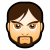判断一个数是否是4个连续整数的和

wwwmmm 发布于 2012/06/19 20:31

年薪达不到25W大数据工程师、拿不到Offer全额退款->>>Given a positive integer, tell whether it is equal to the sum of four consecutive integers.

Input

A positive integer(<231), each case will be on a separate line. A zero (0) denotes the end of input.

Output

The phrase “ is not the sum of four consecutive integers.” or the phrase “ is the sum of four consecutive integers.”, where is the number in question.

Example

In this example, 2 = -1 + 0 + 1 + 2, and 82 = 19 + 20 + 21 + 22. 5 and 41 are not of the correct form.

main(){
int a;
int b;
printf("输入一个整数 a\n");
scanf("%d",&a);
b=(a-10)/4;
if(b==(int)(b)){
printf("%d 是四个连续整数的和\n",b);
}else{
printf("%d 不是四个连续整数的和\n",b);
}
}

0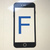n/2 整除， n/2>4, n/2%2==1  满足这些条件就行了

n,n+1,n+2,n+3 , n (1...n)

n+n+1+n+2+n+3 = 4n+6 = 2(n+3)

n+1+n+2 == n+n+3 (5...2n+3)

2n+3 为奇数

0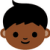0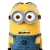引用来自“星迷”的答案

0引用来自“星迷”的答案

0引用来自“星迷”的答案

0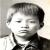if(b==(int)(b)){

0int getX(unsigned int i){
if ((i & 0x3) == 2){
printf("the %d is %d * 4 + 6!\n",i,(i - 6)>>2); return 0;
}else{
printf("shit ! what's this!\n");return 1;
}

}

0引用来自“中山野鬼”的答案

int getX(unsigned int i){
if ((i & 0x3) == 2){
printf("the %d is %d * 4 + 6!\n",i,(i - 6)>>2); return 0;
}else{
printf("shit ! what's this!\n");return 1;
}

}

0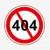0